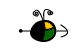Rate Laws from Rate Versus Concentration Data (Differential Rate Laws)

A differential rate law is an equation of the formIn order to determine a rate law we need to find the values of the exponents n, m, and p, and the value of the rate constant, k.

Determining Exponents for a Rate Law from Reaction Orders

If we are given the reaction orders for a reaction, we have the values of the coefficients we need to write the rate law. For example, if we are told that a reaction is second order in A we know that n is equal to 2 in the rate law.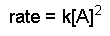Determining Exponents for a Rate Law from Initial Rates (Experimental Data)

If we are given data from two or more experiments at the same temperature with different concentrations of reactants and different rates we can determine the exponents in the differential rate law for the reaction as follows:

1. Write the rate law with the concentrations of all species for which data is given. Write the coefficients as unknowns: n, m, etc. For example, we might have an equation such as the following: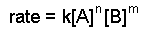2. Take ratios of the experimental data that give different rates.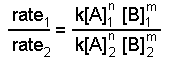3. Cancel common terms and solve for the exponent that does not cancel.

Example

If we have the following experimental initial rate data for the reaction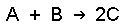experiment [A], M [B], M rate = -d[A]/dt, M h-1 1 0.50 0.50 1.2 2 1.0 0.50 4.8 3 2.0 1.0 38.4

We can write ratios for the data from experiments 1 and 2Using the data from experiments 1 and 2, we see that the k's cancel as do the concentrations of species B.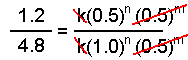Solving this equation for n yields n = 2.

Now we use the known value of n and data from experiments 1 and 3 or from experiments 2 and 3 and solve for m. Here we use experiments 1 and 3: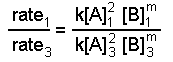When we substitute the data we get: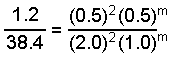Solution of this equation gives m = 1 and the rate law can be written: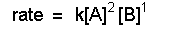Determining k, the Rate Constant

We can determine a rate constant from a differential rate law by substituting a rate and the corresponding concentrations (for example, data from any of the experiments above) into a rate law and solving for k. Using the data from experiments 1, 2, or 3 we could solve the following equation for k: